Overunity theory article

Incompatibility of Planck's Version of the Second Law with

Gibbs's Thermodynamics Regarding Mixtures in Fields

by W.D.Bauer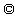released 29.5.96

Abstract:

It is proved that Planck's and Clausius's version of the 2nd law contradict to Gibbs's thermodynamics if cycles of mixtures in fields are included into consideration. Experiments support the Landau-Lifshitz formulation of the 2nd law for fields which allows 100% efficiency conversion of heat into electrical work under isothermal conditions.

1)Introduction

It is well known theory that according to Gibbs's version of the second law the thermodynamic potentials go to an extremum. Gibbs's formulation is regarded as equivalent to Planck's perpetuum mobile forbidding version of the 2nd law although the complete equivalence between both formulations of the second law never have been proved generally, especially if we apply the extremum principle of the thermodynamic potentials to electromagnetic fields. In this article we will investigate this question.

2)The Second Law for Electric and Magnetic Fields

According to Landau and Lifshitz  the free enthalpies G' and G" of a capacitive loaded system are defined by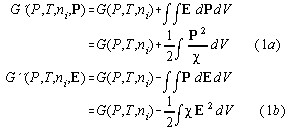where we used the definitions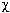=susceptibility, E= electric field, P= electric polarisation, V=volume,and P=pressure. (we omit dielectric constants of vacuum) In differential form we haveRegarding the 2nd derivative with respect to the electric variables P or E of both these potentials we see in formula (1a)+(1b) that for constant homogeneous dielectrics G'(P,T,ni,P) approaches a minimum in the extremal state of thermodynamic equilibrium and G''(P,T,ni,E) approaches a maximum in the equilibrium state, if> 0, dT=0 and dP=0. Therefore, for irreversible changes of state the following unequalities hold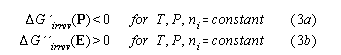We call these formulations of the second law the Landau - Lifshitz versions of the second law. Similar considerations hold for magnetic fields as well.

3) Isothermal Isobaric Electric Cycles of Non-Linear Dielectrics through Phase Transitions

In this section we investigate isothermal and isobaric electrothermic cycle with an intense electric field applied to a non-linear dielectric substance in a capacitor. One part of the cycle is reversible, the other is irreversible. T and P are constant (dT=0,dP=0) during the whole cycle.

It is well known that electric cycles of ferroelectrets show a loss hysteresis of electric work. Using the Landau-Lifshitz version of the 2nd law we will show that we have a hysteretic loss if irreversibilities occur in the cycle at fixed polarisation P. Therefore, touching point 1 and 2 we proceed a closed isothermal isobaric electric cycle (1->2->1) in the P-E -diagram with an irreversible part (2->1) at constant polarisation P . Because G' is a potential it holds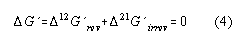The second law is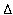G'(2->1)<0. According to our initial assumption we have P1=P2 and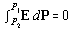during the irreversible phase (2->1) of the cycle. This zero expression is added to the first line of (1a) and using formula (3a) and (4) we obtain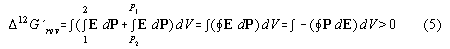Regarding the sign of this integral we see that we have a loss hysteresis of electric work for irreversibilities at constant polarisation P.

An analogous consideration we apply to a cycle with an irreversibity at fixed field E: Because G'' is a potential, for a closed cycle over three points(1->2->3->1), cf. fig.2, we have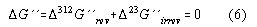According to the Landau-Lifshitz version of the 2nd lawG"irrev > 0 holds because G"irrev goes to a maximum. Because the of isofield (E2=E3) irreversible change of state(2->3) we have also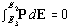. This zero expression is added to the first line of (1b) and using formula (3b) and (6) we can write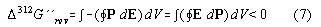Regarding the sign of this integral we see that the hysteresis of this cycle is reversed compared to the usual hysteresis of a ferroelectric substance. This is equivalent to an electric cycle with only one heat reservoir which changes the heat of the environment to work. The proof is as follows: If a closed cycle of the internal energy U is proceeded the equation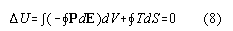holds because U is a potential. Therefrom, because the first term is negative, it follows that the net heat exchange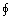T dS has a positive sign. This impliesdS>0 because T=constant which is contrary to the Clausius formulation of the 2nd law. This means that the cycle takes heat from the environment and gives off electrical work under isothermal conditions.

4) A possible example for violation of Planck's version of second law

As a candidate for realisation of such an effect we propose here a "splitted" isothermal cycle in fields using near critical point solutions investigated and characterized by Wirtz and Fuller . They investigated electrically induced phase transitions of polymer solutions like polystyrene in cyclohexane (upper critical point solution) or p-chlorostyrene in ethylcarbitol (lower critical point solution). The closed splitted cycle is proceeded using such a sol-gel liquid dielectric substance in a capacitor. The composition of the liquid is separated periodically by a demixing phase transitions induced by switching off the field. After the separation of both phases they are remixed again (irreversible phase !) after switching on the strong field.

The cycle is started in the 2- phase region at zero field at the points 1, comp.fig. 1,2 and 3, where the volume is splitted by closing the tap separating both phases. Then a strong electric field E is applied. So we reach the points 2' and 2'' representing different phases of the solution in both compartments. Then we open the tap and let mix the solutions in both compartments. During the mixing (2->3) the electric field is kept constant by decharging the capacitor during the decline of the dieleletric constant, cf. fig.4. In the phase diagram fig. 3 from [2,6] and as well in fig. 1 +2 the mixed solution is at the phase separation line at point 3. Then doing the last step of the cycle the capacitor is decharged completely and the system goes back into the 2-phase area to point 1 and demixes. According to the theory (cf. formula (3b) and (6))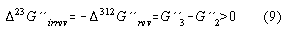Now we define S:=V'/V to be the splitting factor of the total volume V , V' and V''are the volumes of the compartments each with V:=V'+V". We write the difference of the free enthalpy using our definitions assuming:=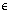-1 to be dependent from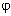and independent from E for zeroth order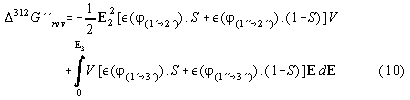The right side of the first line represents the stored linear combined field energy of the separated volume compartments (points 2' and 2") at point 2, the second line stands for the field energy (1->3) of both the connected compartments containing the volume parts of two coexisting phases ' and ". In the first line S,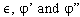are fixed, in the second line S,are dependent from E in 2-phase area. Therefore, if we have a constitutive relation describing the material behaviour we can predict the work gain of the cycle numerically by calculating the phase diagram of the solution. For the system investigated a Flory free-energy density approach of an incompressible dilute monodisperse polymer solution is useful. The "ansatz" is [2,4,5,6]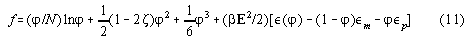where N:= polymerisation number, E:= electric field,is the total dielectric constant,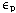is the dielectric constant of the polymer solution,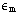is the dielectric constant of the pure monomer solution,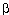:=1/(kT) with k:=Boltzmann number, and:=Flory parameter. Therefrom, the chemical potential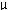and osmotic pressurefollow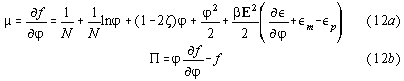The phase equilibrium is determined by the equations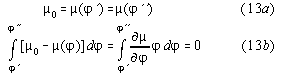The first equation describes the chemical potential to be equal in both phases.The second equation is the Maxwell construction applied to the chemical potential. The solution of this system of equations can be done numerically or by deriving a parameter representation[5,6]. Results are shown qualitatively in fig.3 .

5) Conclusion

We have shown that the second law in the Planck version or in the version of the maximum entropy principle can lead to contradictions to the Gibbs formalism if the influence of fields on irreversible processes is considered. It is dependent from the path of the irreversibility in the state space whether we get a loss or a gain hysteresis of the electric work. Therefore the question arises how to reformulate the second law. Remembering that thermodynamics can be regarded as mechanics of a many-particle system reduced in the number of coordinates then it is clear that a reformulation should include mechanics as well. It is not a new idea that the second law is a consequence of the principle of least action  in mechanics and if we go back to Landau and Lifshitz  we realize that they use the variation principle as well in order to derive the electromagnetic extensions of the thermodynamic potentials from the Maxwell equations.

References:

1)L.D. Landau, E.M.Lifshitz Electrodynamics of Continous Media (Pergamon, Oxford) 1984

2)D. Wirtz, G.G. Fuller Phys.Rev.Lett.71 (1993) 2236

3)P. Debye, K. J. Kleboth J. Chem.Phys.42 (1965) 3155

4)P.G. de Gennes, Scaling Concepts in Polymer Physics (Cornell University Press Ithaca, London 1985)

5)J. Des Cloizeaux ,G. Jannink Polymers in Solution Oxford University Press Oxford 1987

6)D. Wirtz, K. Berend, G.G. Fuller Macromolecules 25 (1992) 7234

7)W.G. Hoover Phys.Lettr. A204 (1995) p.133-135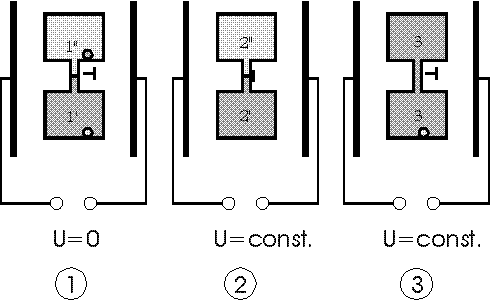Fig 1: Isothermic isobaric electric cycle with a special polymer solution as dielectric 1) voltage U=0: system in 2-phase region 2) both volumes separated, rise of voltage from zero to U=const.: every volume part in 1-phase region 3. 3 ) voltage U=const.: opening the tap and returning to the phase separation line by remixing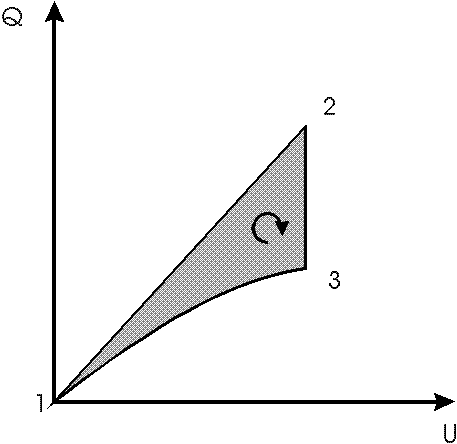Fig.2: isothermal electric cycle in capacitor with electrically induced phase transitions; charge Q versus voltage U plotted; 1 starting at 2 phase area line with zero field, 1-2 applying a field with tap closed, 2 opening the tap, 2-3 discharging and remixing in field, 3 returning to starting point 1 by discharging the capacitor; a negative work area is predicted according to Gibbs thermodynamics contrary to Planck's version of the second law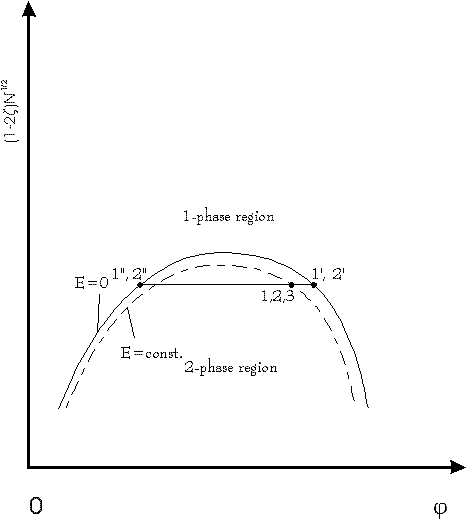Fig.3: phase diagram of a polymer solution with and without electric field E according to ; plot shows modified Flory-parameter versus volume fraction of polymers; points 1: E=0, 2-phases, both points 1 at the phase separation line; points 2: E=const., both points 2 of the splitted volume in 1 phase area; point 3: E=const., after opening the tap: point 3 return exactly at the phase separation line; more information about the construction of this phase diagrams, see references [5,6]Fig.4: Dielectric constantversus volume fractionof polymers in a dilute solution; points 1, 2 and 3 refer to points 1-3 in fig. 1 -3. According to the theory [2,6]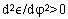holds near the critical point. Therefore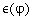has to turn to the left and the dielecric constant has to decline during remixing 2->3. Observations at a similar system  support this prediction